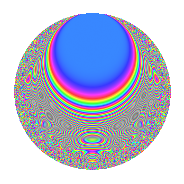Properties

 Label 8041.2.a.hLevel 8041 Weight 2 Character orbit 8041.a Self dual Yes Analytic conductor 64.208 Analytic rank 1 Dimension 74 CM No

Related objects

Newspace parameters

 Level: $$N$$ = $$8041 = 11 \cdot 17 \cdot 43$$ Weight: $$k$$ = $$2$$ Character orbit: $$[\chi]$$ = 8041.a (trivial)

Newform invariants

 Self dual: Yes Analytic conductor: $$64.2077082653$$ Analytic rank: $$1$$ Dimension: $$74$$ Fricke sign: $$1$$ Sato-Tate group: $\mathrm{SU}(2)$

$q$-expansion

The dimension is sufficiently large that we do not compute an algebraic $$q$$-expansion, but we have computed the trace expansion.

 $$\operatorname{Tr}(f)(q) =$$ $$74q$$ $$\mathstrut -\mathstrut 7q^{2}$$ $$\mathstrut -\mathstrut 3q^{3}$$ $$\mathstrut +\mathstrut 79q^{4}$$ $$\mathstrut -\mathstrut 6q^{5}$$ $$\mathstrut -\mathstrut 12q^{6}$$ $$\mathstrut -\mathstrut 16q^{7}$$ $$\mathstrut -\mathstrut 21q^{8}$$ $$\mathstrut +\mathstrut 75q^{9}$$ $$\mathstrut +\mathstrut O(q^{10})$$ $$\operatorname{Tr}(f)(q) =$$ $$74q$$ $$\mathstrut -\mathstrut 7q^{2}$$ $$\mathstrut -\mathstrut 3q^{3}$$ $$\mathstrut +\mathstrut 79q^{4}$$ $$\mathstrut -\mathstrut 6q^{5}$$ $$\mathstrut -\mathstrut 12q^{6}$$ $$\mathstrut -\mathstrut 16q^{7}$$ $$\mathstrut -\mathstrut 21q^{8}$$ $$\mathstrut +\mathstrut 75q^{9}$$ $$\mathstrut -\mathstrut 9q^{10}$$ $$\mathstrut -\mathstrut 74q^{11}$$ $$\mathstrut -\mathstrut 3q^{12}$$ $$\mathstrut +\mathstrut 4q^{13}$$ $$\mathstrut -\mathstrut 5q^{14}$$ $$\mathstrut -\mathstrut 17q^{15}$$ $$\mathstrut +\mathstrut 85q^{16}$$ $$\mathstrut -\mathstrut 74q^{17}$$ $$\mathstrut -\mathstrut 23q^{18}$$ $$\mathstrut -\mathstrut 21q^{20}$$ $$\mathstrut -\mathstrut 22q^{21}$$ $$\mathstrut +\mathstrut 7q^{22}$$ $$\mathstrut -\mathstrut 23q^{23}$$ $$\mathstrut -\mathstrut 51q^{24}$$ $$\mathstrut +\mathstrut 90q^{25}$$ $$\mathstrut -\mathstrut 46q^{26}$$ $$\mathstrut -\mathstrut 27q^{27}$$ $$\mathstrut -\mathstrut 61q^{28}$$ $$\mathstrut -\mathstrut 63q^{29}$$ $$\mathstrut -\mathstrut 22q^{30}$$ $$\mathstrut -\mathstrut 31q^{31}$$ $$\mathstrut -\mathstrut 69q^{32}$$ $$\mathstrut +\mathstrut 3q^{33}$$ $$\mathstrut +\mathstrut 7q^{34}$$ $$\mathstrut -\mathstrut 20q^{35}$$ $$\mathstrut +\mathstrut 51q^{36}$$ $$\mathstrut +\mathstrut 8q^{37}$$ $$\mathstrut -\mathstrut 2q^{38}$$ $$\mathstrut -\mathstrut 77q^{39}$$ $$\mathstrut -\mathstrut 37q^{40}$$ $$\mathstrut -\mathstrut 64q^{41}$$ $$\mathstrut +\mathstrut 13q^{42}$$ $$\mathstrut -\mathstrut 74q^{43}$$ $$\mathstrut -\mathstrut 79q^{44}$$ $$\mathstrut -\mathstrut 12q^{45}$$ $$\mathstrut -\mathstrut 53q^{46}$$ $$\mathstrut -\mathstrut 32q^{47}$$ $$\mathstrut +\mathstrut 2q^{48}$$ $$\mathstrut +\mathstrut 78q^{49}$$ $$\mathstrut -\mathstrut 104q^{50}$$ $$\mathstrut +\mathstrut 3q^{51}$$ $$\mathstrut +\mathstrut 13q^{52}$$ $$\mathstrut +\mathstrut 25q^{53}$$ $$\mathstrut -\mathstrut 110q^{54}$$ $$\mathstrut +\mathstrut 6q^{55}$$ $$\mathstrut -\mathstrut 29q^{56}$$ $$\mathstrut -\mathstrut 29q^{57}$$ $$\mathstrut -\mathstrut 14q^{58}$$ $$\mathstrut -\mathstrut 61q^{59}$$ $$\mathstrut -\mathstrut 82q^{60}$$ $$\mathstrut -\mathstrut 36q^{61}$$ $$\mathstrut -\mathstrut 63q^{62}$$ $$\mathstrut -\mathstrut 104q^{63}$$ $$\mathstrut +\mathstrut 107q^{64}$$ $$\mathstrut -\mathstrut 65q^{65}$$ $$\mathstrut +\mathstrut 12q^{66}$$ $$\mathstrut +\mathstrut 33q^{67}$$ $$\mathstrut -\mathstrut 79q^{68}$$ $$\mathstrut -\mathstrut 34q^{69}$$ $$\mathstrut -\mathstrut 3q^{70}$$ $$\mathstrut -\mathstrut 168q^{71}$$ $$\mathstrut -\mathstrut 67q^{72}$$ $$\mathstrut -\mathstrut 47q^{73}$$ $$\mathstrut -\mathstrut 54q^{74}$$ $$\mathstrut -\mathstrut 53q^{75}$$ $$\mathstrut -\mathstrut 4q^{76}$$ $$\mathstrut +\mathstrut 16q^{77}$$ $$\mathstrut -\mathstrut 3q^{78}$$ $$\mathstrut -\mathstrut 79q^{79}$$ $$\mathstrut -\mathstrut 59q^{80}$$ $$\mathstrut +\mathstrut 70q^{81}$$ $$\mathstrut -\mathstrut 18q^{82}$$ $$\mathstrut -\mathstrut 36q^{83}$$ $$\mathstrut -\mathstrut 118q^{84}$$ $$\mathstrut +\mathstrut 6q^{85}$$ $$\mathstrut +\mathstrut 7q^{86}$$ $$\mathstrut -\mathstrut 24q^{87}$$ $$\mathstrut +\mathstrut 21q^{88}$$ $$\mathstrut -\mathstrut 24q^{89}$$ $$\mathstrut +\mathstrut 25q^{90}$$ $$\mathstrut -\mathstrut 14q^{91}$$ $$\mathstrut -\mathstrut 18q^{92}$$ $$\mathstrut -\mathstrut 13q^{93}$$ $$\mathstrut +\mathstrut 9q^{94}$$ $$\mathstrut -\mathstrut 155q^{95}$$ $$\mathstrut -\mathstrut 50q^{96}$$ $$\mathstrut +\mathstrut q^{97}$$ $$\mathstrut -\mathstrut 60q^{98}$$ $$\mathstrut -\mathstrut 75q^{99}$$ $$\mathstrut +\mathstrut O(q^{100})$$

Embeddings

For each embedding $$\iota_m$$ of the coefficient field, the values $$\iota_m(a_n)$$ are shown below.

For more information on an embedded modular form you can click on its label.

Label $$a_{2}$$ $$a_{3}$$ $$a_{4}$$ $$a_{5}$$ $$a_{6}$$ $$a_{7}$$ $$a_{8}$$ $$a_{9}$$ $$a_{10}$$
1.1 −2.77959 −0.489198 5.72614 −0.717152 1.35977 −1.78399 −10.3571 −2.76069 1.99339
1.2 −2.77350 −1.80573 5.69228 3.84925 5.00818 −3.56425 −10.2405 0.260654 −10.6759
1.3 −2.72314 3.10551 5.41549 −3.61264 −8.45674 −4.38477 −9.30085 6.64419 9.83772
1.4 −2.65828 2.25599 5.06647 2.74441 −5.99706 1.84456 −8.15154 2.08950 −7.29541
1.5 −2.63295 1.21952 4.93245 −0.402998 −3.21093 4.56516 −7.72100 −1.51278 1.06108
1.6 −2.60873 −2.96905 4.80548 −3.25113 7.74546 1.59414 −7.31874 5.81527 8.48133
1.7 −2.58183 0.610196 4.66583 −3.25776 −1.57542 −0.634845 −6.88270 −2.62766 8.41096
1.8 −2.56683 −0.396689 4.58863 1.36693 1.01823 2.28939 −6.64457 −2.84264 −3.50868
1.9 −2.38029 3.05182 3.66578 3.97493 −7.26423 −3.90026 −3.96504 6.31363 −9.46150
1.10 −2.34491 −2.19404 3.49862 1.59097 5.14484 −2.37576 −3.51413 1.81381 −3.73069
1.11 −2.21900 −1.42344 2.92394 −3.24388 3.15861 4.34231 −2.05022 −0.973808 7.19815
1.12 −2.15071 2.55470 2.62555 −0.0279293 −5.49442 −2.62385 −1.34537 3.52651 0.0600678
1.13 −2.14896 −2.65732 2.61805 2.21050 5.71048 0.288836 −1.32816 4.06133 −4.75028
1.14 −2.02657 2.55915 2.10699 −3.25953 −5.18630 3.04044 −0.216828 3.54925 6.60567
1.15 −1.90535 −0.951044 1.63036 −1.53040 1.81207 −2.50310 0.704287 −2.09552 2.91594
1.16 −1.89916 −0.231765 1.60683 2.27252 0.440160 0.501161 0.746704 −2.94628 −4.31590
1.17 −1.85483 0.655235 1.44041 4.10800 −1.21535 −1.24342 1.03795 −2.57067 −7.61965
1.18 −1.83407 2.68858 1.36381 −0.219056 −4.93104 2.19442 1.16682 4.22847 0.401763
1.19 −1.66618 −0.454715 0.776164 0.422618 0.757638 −4.38423 2.03913 −2.79323 −0.704158
1.20 −1.65992 0.748300 0.755318 −4.19197 −1.24211 −4.84394 2.06607 −2.44005 6.95831
See all 74 embeddings
 $$n$$: e.g. 2-40 or 990-1000 Embeddings: e.g. 1-3 or 1.74 Significant digits: Format: Complex embeddings Normalized embeddings Satake parameters Satake angles

Inner twists

This newform does not have CM; other inner twists have not been computed.

Atkin-Lehner signs

$$p$$ Sign
$$11$$ $$1$$
$$17$$ $$1$$
$$43$$ $$1$$

Hecke kernels

This newform can be constructed as the kernel of the linear operator $$T_{2}^{74} + \cdots$$ acting on $$S_{2}^{\mathrm{new}}(\Gamma_0(8041))$$.